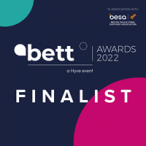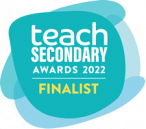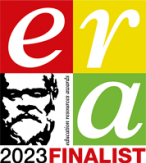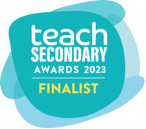# OCR A level (H046-H446) SLR 13 – Data Types

This download provides you with a zip file which contains all the resources you will need to deliver this topic.

It includes:

• Student Learning Record (A3 version in word)
• Student Learning Record (PowerPoint version)
• Student Learning Record (Exemplars)
• Set of student activities and workshops with answers
• Answers to the exam questions from the back of the SLR
• Teacher Marking Checklist
• Year 13 Recap Lesson

This topic covers:

H046

• 1.4.1 a-h Data Types

H446

• 1.4.1 a-j Data Types

Specific knowledge required for AS and A Level:

• Candidates need to have an understanding of programming data types such as integer, real, Boolean, character, string etc.
• Candidates need to be able to choose appropriate data types for a situation or given data.
• Candidates should have experience of programming solutions using these data types.
• Candidates should have knowledge of how to convert from one data type to another (casting).
• Candidates should understand how and why computers store data as binary, and that a binary number can have a variety of different interpretations depending on what is being stored (e.g. numeric, text, image, sound).
• Candidates should be able to convert positive whole numbers to binary and from binary to denary.
• Candidates should know how to store negative numbers using Sign and Magnitude and Two’s Complement.
• Candidates should be able to convert denary numbers to sign and magnitude, and two’s complement – and vice-versa.
• Candidates should be able to perform addition and subtraction on integer binary numbers. (These numbers could be positive or negative using two’s complement representation.)
• Candidates need to have an understanding of the purpose and potential uses of hexadecimal for example where and why they are used instead of binary and the benefits of using hexadecimal over alternatives such as binary.
• Candidates should be able to convert denary numbers to hexadecimal and vice-versa and from binary to hexadecimal and vice-versa.
• Candidates should have an understanding of how (positive and negative) real numbers are represented in a binary floating-point representation, and should be able to convert between a denary number and a real binary number. (NB the representation used for the exam is the mantissa and exponent both represented using two’s complement.)
• Candidates should understand the need for normalised floating point numbers.
• Candidates should be able to normalise a floating point number.
• Candidates should have an understanding of how characters are represented in binary.
• Candidates should understand the need for a character set and how a computer makes use of a character set.
• Candidates should be aware of the ASCII and UNICODE character sets and be able to explain the differences between these and the benefits of each.
• Candidates should be able to use a character set, or part of a character set, to translate characters into binary and vice-versa. (Candidates are not expected to memorise any values in a character set)

Specific knowledge required for A Level ONLY:

• Candidates should be able to normalise a floating point number. Candidates should be able to perform addition and subtraction floating point arithmetic including addition and subtraction of both positive and negative numbers.
• Candidates should be able to perform right and left logical shifts.
• Candidates should understand the effect of right and left shifts on a binary numbers.
• Candidates should understand the purpose of using masks with bitwise operators, and should have experience of applying masks using AND, OR and XOR.Registered in England and Wales: 10442992

VAT Number: 290 9845 58

Telephone: 020 4519 3010